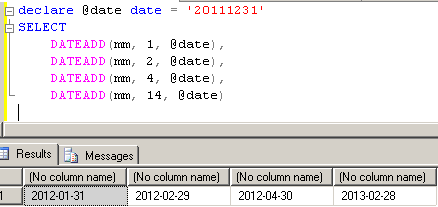﻿ SQL Server DateAdd Function T-SQL ExampleSQL Server and T-SQL Development Tutorials Development resources, articles, tutorials, code samples, tools and downloads for ASP.Net, SQL Server, Reporting Services, T-SQL, Windows, AWS, SAP HANA and ABAP

# SQL Server DateAdd Function T-SQL Example

SQL Server DateAdd function returns date which is added a time inverval identified by "basic datetime interval units times a given number of times" to a specific input date or datetime parameter.

T-SQL developers can understand the above complex sql dateadd function definition by looking at the below T-SQL DATEADD syntax.

The SQL Server DATEADD function takes 3 arguments:
DATEPART is used the unit of time interval which will used during tsql DATEADD function,
NUMBER is used to set the time span with the help of DATEPART argument, which will be added to the base datetime value,
DATE is the base datetime parameter value, the time span will be added to this DATE argument or will be substracted from this DATE argument.

The output of the SQL Server DateAdd function is just as the input Date argument sql data type.
If the Date argument is in sql DateTime data type, the SQL Server DateAdd function output is also in DateTime.
If the Date argument is in tsql Date data type, the DateAdd function return value is also in sql Date data type.
All possible SQL Server date and time data types are datetime, smalldatetime, date, time, datetime2, and datetimeoffset

Let's set up a basic sql DATEADD scenario in a SQL Server database platform.
Assume that you have a table which stores service desk requests. And this table has InsertDate column. There is a business requirement which requires you to create a control after 3 days later the InsertDate column value.
So you decide to add a new table column storing the datetime value which is 3 days later than the InsertDate column.
In the INSTEAD OF INSERT trigger, or the Computed Column will have a t-sql expression using SQL Server DateAdd shown like below :

It is obvious that if the t-sql developer wants to substract a certain time span then the Number argument should be a Negative value in the SQL Server DateAdd function.

In order to use SQL Server DATEADD function extensively, a t-sql developer can use the following DATEADD DATEPART arguments.

 DATEPART Argument (unit of time) DATEADD DATEPART Abbreviation Year YY, YYYY Quarter Q, QQ Month M, MM DayOfYear DY, Y Day DD, D Week WK, WW Weekday DW, W Hour HH Minute MI, N Second S, SS Millisecond MS Microsecond MCS Nanosecond NS

I can easily say that the most common used DATEPART DATEADD arguments are Year (yy), Month (mm), Day (dd), Hour (hh), and Minute (mi).
As a t-sql developer, if you memorize these DatePart abbreviations, it will be very easy for you to use SQL Server DateAdd function in your sql queries or sql scripts.

Please note that the Number argument in SQL Server DateAdd datetime function is in integer.
So is a value bigger than 2147483647 or smaller than -2147483648 will cause an arithmetic overflow error.

One important point with SQL Server DATEADD function while using with MONTH interval is the end dates of the months.
For example, if the base date is the 31st of the month, then if one month is added using t-sql DATEADD, the result will be 31st, 30th or 28th (maybe 29th) of the following month.
Let's make a sample.Here below, t-sql developers can find SQL Server DateAdd function examples with different date and time data types as argument to the DateAdd function.

If the date argument data type and the time interval argument is not compatible, the sql engine will throw an sql exception like :

select